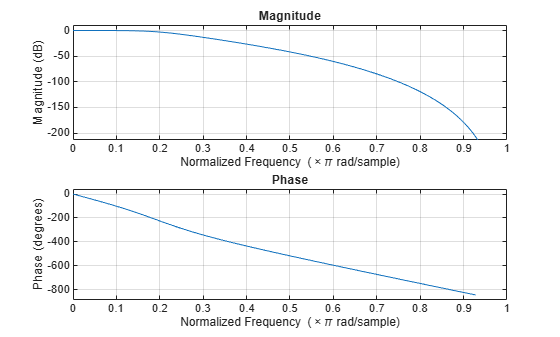# maxflat

Generalized digital Butterworth filter design

## Syntax

```[b,a] = maxflat(n,m,Wn) b = maxflat(n,'sym',Wn) [b,a,b1,b2] = maxflat(n,m,Wn) [b,a,b1,b2,sos,g] = maxflat(n,m,Wn) [...] = maxflat(n,m,Wn,'design_flag') ```

## Description

`[b,a] = maxflat(n,m,Wn)` is a lowpass Butterworth filter with numerator and denominator coefficients `b` and `a` of orders `n` and `m`, respectively. `Wn` is the normalized cutoff frequency at which the magnitude response of the filter is equal to $1/\sqrt{2}$ (approximately –3 dB). `Wn` must be between 0 and 1, where 1 corresponds to the Nyquist frequency.

`b = maxflat(n,'sym',Wn)` is a symmetric FIR Butterworth filter. `n` must be even, and `Wn` is restricted to a subinterval of [0,1]. The function raises an error if `Wn` is specified outside of this subinterval.

`[b,a,b1,b2] = maxflat(n,m,Wn)` returns two polynomials `b1` and `b2` whose product is equal to the numerator polynomial `b` (that is, `b = conv(b1,b2)`). `b1` contains all the zeros at `z` = `-1`, and `b2` contains all the other zeros.

`[b,a,b1,b2,sos,g] = maxflat(n,m,Wn)` returns the second-order sections representation of the filter as the filter matrix `sos` and the gain `g`.

`[...] = maxflat(n,m,Wn,'design_flag')` enables you to monitor the filter design, where `'design_flag'` is

• `'trace'` for a textual display of the design table used in the design

• `'plots'` for plots of the filter's magnitude, group delay, and zeros and poles

• `'both'` for both the textual display and plots

## Examples

collapse all

Design a generalized Butterworth filter with normalized cutoff frequency $0.2\pi$ rad/s. Specify a numerator order of 10 and a denominator order of 2. Visualize the frequency response of the filter.

```n = 10; m = 2; Wn = 0.2; [b,a] = maxflat(n,m,Wn); fvtool(b,a)```## Algorithms

The method consists of the use of formulae, polynomial root finding, and a transformation of polynomial roots.

## References

 Selesnick, Ivan W., and C. Sidney Burrus. “Generalized Digital Butterworth Filter Design.” IEEE® Transactions on Signal Processing. Vol. 46, Number 6, 1998, pp. 1688–1694.